NEET  >  Test: Equilibrium of Rigid Bodies

# Test: Equilibrium of Rigid Bodies

Test Description

## 10 Questions MCQ Test Physics Class 11 | Test: Equilibrium of Rigid Bodies

Test: Equilibrium of Rigid Bodies for NEET 2022 is part of Physics Class 11 preparation. The Test: Equilibrium of Rigid Bodies questions and answers have been prepared according to the NEET exam syllabus.The Test: Equilibrium of Rigid Bodies MCQs are made for NEET 2022 Exam. Find important definitions, questions, notes, meanings, examples, exercises, MCQs and online tests for Test: Equilibrium of Rigid Bodies below.
Solutions of Test: Equilibrium of Rigid Bodies questions in English are available as part of our Physics Class 11 for NEET & Test: Equilibrium of Rigid Bodies solutions in Hindi for Physics Class 11 course. Download more important topics, notes, lectures and mock test series for NEET Exam by signing up for free. Attempt Test: Equilibrium of Rigid Bodies | 10 questions in 10 minutes | Mock test for NEET preparation | Free important questions MCQ to study Physics Class 11 for NEET Exam | Download free PDF with solutions
 1 Crore+ students have signed up on EduRev. Have you?
Test: Equilibrium of Rigid Bodies - Question 1

### Arrange the comparative stabilities of the three objects shown in the figure in terms of work and potential energy.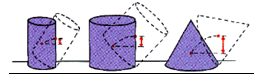Detailed Solution for Test: Equilibrium of Rigid Bodies - Question 1

A object’s stability depends upon its centre of gravity.the larger the centre of gravity the more stable,By looking at the figures we can easily figure out which is more stable.

Test: Equilibrium of Rigid Bodies - Question 2

### The figure shows a dish kept at an orientation with a marble placed on it. If the marble in figure is displaced slightly, it would rattle about for a while and come to rest in its original place. This is an example of :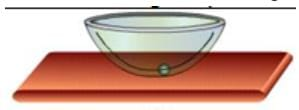Detailed Solution for Test: Equilibrium of Rigid Bodies - Question 2

If a dish is kept at an orientation with a marble placed on it and if the marble in figure is displaced slightly, it would rattle about for a while and come to rest in its original place.  This is the example of Stable Equilibrium.
When a body tries to regain its equilibrium position after being slightly displaced and released, it is said to be in stable equilibrium.

Test: Equilibrium of Rigid Bodies - Question 3

### The rock has a mass of 1 kg. What is the mass of the measuring stick if it is balanced by a support force at the one-quarter mark? The centre of mass of the measuring stick is at the one-half mark, one-quarter of the stick’s length beyond the pivot.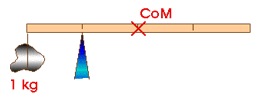Detailed Solution for Test: Equilibrium of Rigid Bodies - Question 3

The stick is balanced by the 1 kg rock that is one-quarter of its length to the left and by its own weigh which is at the Centre of Mass, one-quarter of its length to the right.  Therefore, the mass of the stick must be one kilogram, 1 kg.

Test: Equilibrium of Rigid Bodies - Question 4

A pair of equal and opposite forces with different line of action are said to form a

Detailed Solution for Test: Equilibrium of Rigid Bodies - Question 4

When two forces of equal magnitude opposite in direction and acting along parallel straight lines, then they are said to form a couple. The perpendicular distance between the two force forming a couple is called the arm of the couple.

Test: Equilibrium of Rigid Bodies - Question 5

A meter rule is supported at its centre. It is balanced by two weights, A and B. If A and B are placed at a distance 20 cm, and 40 cm from the centre of scale, find the weight of B. The weight of A is 50N.

Detailed Solution for Test: Equilibrium of Rigid Bodies - Question 5

In balanced condition torque of the both sides must be equal so Fa × Da = Fb × Db
50 × 20 = Fb × 40
Fb = 25 N

Test: Equilibrium of Rigid Bodies - Question 6

In order to rotate a body, we need to apply

Detailed Solution for Test: Equilibrium of Rigid Bodies - Question 6

In order to rotate a body, we need to apply two equal forces at two ends but in the opposite direction.

Test: Equilibrium of Rigid Bodies - Question 7

Determine the force that must be applied to end of the class 1 lever shown below to lift the 500 lb load.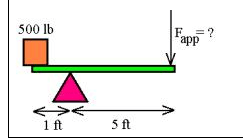Detailed Solution for Test: Equilibrium of Rigid Bodies - Question 7

Balance the 2 opposite moments of inertia on the 2 sides
1 × 500 = F × 5
F = 100

Test: Equilibrium of Rigid Bodies - Question 8

Five forces are separately applied to a flat object lying on a table of negligible friction as shown in figure. The force which will not cause the object to rotate about the centre of the object is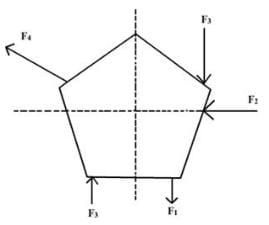Detailed Solution for Test: Equilibrium of Rigid Bodies - Question 8

The line of action of F2 passes through the centre of rotation, and thus cannot produce a torque about the centre (r = 0).

Test: Equilibrium of Rigid Bodies - Question 9

Which of the following appliance works on the principle of moments.

Detailed Solution for Test: Equilibrium of Rigid Bodies - Question 9

In beam balance we consider the torque at centre of beam which is moment of force at its each end.

Test: Equilibrium of Rigid Bodies - Question 10

There are two blocks of mass 3 kg and 5 kg hanging from the ends of a rod of negligible mass. The rod is marked in eight equal parts as shown. At which of the points indicated should a string be attached if a rod is to remain horizontal when suspended from the string?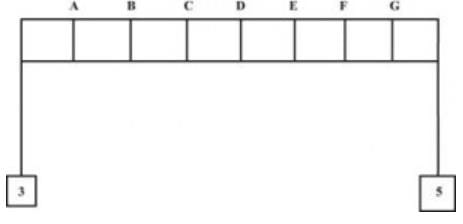Detailed Solution for Test: Equilibrium of Rigid Bodies - Question 10

This is based on torque balance, That is F.d=const.
Therefore 3×d=5×(8-d)
by solving we get d=5.
and at point 5=E

## Physics Class 11

126 videos|449 docs|210 tests
 Use Code STAYHOME200 and get INR 200 additional OFF Use Coupon Code
Information about Test: Equilibrium of Rigid Bodies Page
In this test you can find the Exam questions for Test: Equilibrium of Rigid Bodies solved & explained in the simplest way possible. Besides giving Questions and answers for Test: Equilibrium of Rigid Bodies, EduRev gives you an ample number of Online tests for practice

## Physics Class 11

126 videos|449 docs|210 tests

### How to Prepare for NEET

Read our guide to prepare for NEET which is created by Toppers & the best Teachers